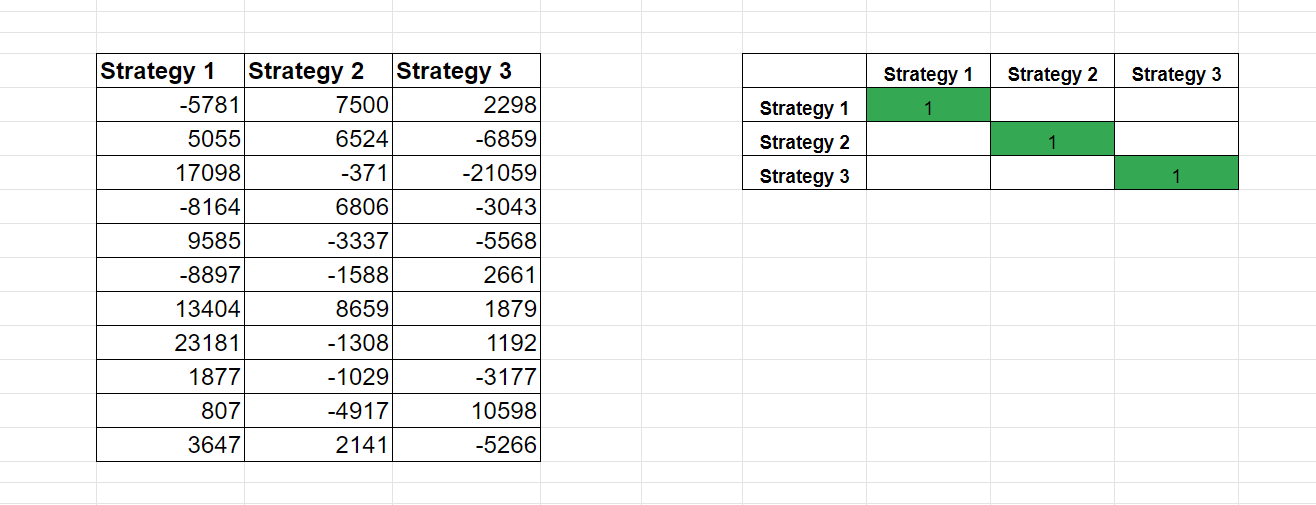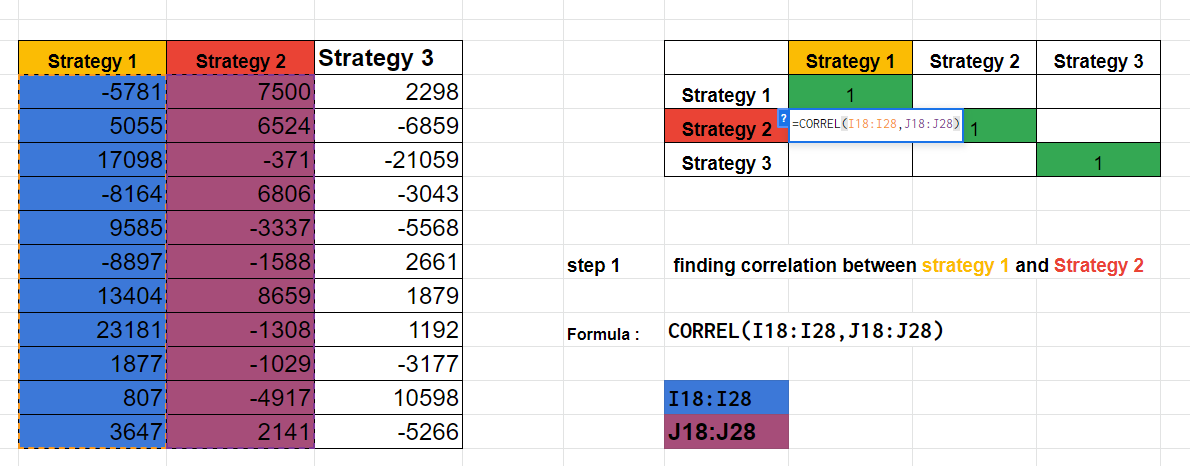# Finding Correlation between strategies

4.5
(13)

We all know that the Stock market is random and it has Three-phase cycles:

1. Uptrend
2. Downtrend
3. Sideways

Do you really think your strategy will perform really well in all market conditions?

Really Big No……….

As we all know, It is hard to find a strategy that will work well in all market conditions.

So that means you have to go through the Drawdown Phases of strategy.

What will it cause?

It will affect your psychology. If it has higher Drawdowns periods, maybe you will stop your strategy thinking that it is not working before it hits Maximum Drawdowns.

What is the solution?

I will try to find multiple diversified Strategies.

What does that mean?

In simple words, I will try to find a Basket of strategies that will work well in different market conditions. i.e Some strategies will work well in sideways market conditions, some will work well at uptrend market conditions and some will be good at downtrend market conditions.

How will this help us?

Because you are running diversified strategies, your Drawdown will reduce tremendously and It will not affect your psychology, which will help you to trade all of the strategies for long the run.

Now, a question must be running in your mind, If something is running well in one market condition and another is giving loss then will I end up playing a zero-sum game?

For this, we are going to analyze no-correlation strategies with zero or near to zero correlation with each other, which means their behavior is independent not the same or opposite.

We get the idea that this problem can be solved by finding the correlation between strategies.

What is correlation?

It’s a statistical term that is used to measure relations between two Data sets.

These two Data sets can be anything like D-mart and Nifty-50 Behavior or it maybe BankNifty and Nifty-50.

It’s hard to predict correlation because these are constantly changing values and mostly during panic and high volatility regimes when most assets are moving downward directions.

So, Correlation ranges between -1 to 1.

• Consider 2 Stocks If they are moving in the same direction, then they will have positive correlation between 0 to 1 and If they are moving exactly the same then it has a correlation value of +1.
• If they are moving in opposite directions, then they will have Negative Correlation between 0 to -1 and If they are moving exactly opposite then it has a correlation value of -1.
• If they are moving independent of each other, they will have a correlation value of 0.

Now, we will find a correlation between strategies using their historic PNL data and how they are correlated.

As we are going to find a correlation between multiple strategies :

The matrix method will be used to find correlations between strategies. If we have N strategies then we are going to use the ( N x N ) matrix to find a correlation between them.

I am going to use Python to find correlation and Data samples will be strategies PNL.

Step 1: Collect the data ( Let’s Take 3 strategies PNL )

You can take this as PNL as Weekly, Monthly or Yearly

Step 2 : Created Dataframe using Pandas library

Code:

Import pandas as pd

data = {‘strategy_1’: [-5781, 5055, 17098, -8164, 9585, -8897, 13404, 23181, 1877, 807, 3647],

‘strategy_2’: [7500, 6524, -371, 6806, -3337, -1588, 8659, -1308, -1029, -4917, 2141],

‘strategy_3’: [2298, -6859, -21059, -3043, -5568, 2661, 1879, 1192, -3177, 10598, -5266]

}

df = pd.DataFrame(data,columns=[‘strategy_1′,’strategy_2′,’strategy_3’])

print(df)

Result :

Step 3 : Create correlation Matrix using Pandas Library

Use ” df.corr() “ to find correlation

Code :

import pandas as pd

data = {‘strategy_1’: [-5781, 5055, 17098, -8164, 9585, -8897, 13404, 23181, 1877, 807, 3647],

‘strategy_2’: [7500, 6524, -371, 6806, -3337, -1588, 8659, -1308, -1029, -4917, 2141],

‘strategy_3’: [2298, -6859, 21059, -3043, -5568, 2661, 1879, 1192, -3177, 10598, -5266]

}

df = pd.DataFrame(data,columns=[‘strategy_1′,’strategy_2′,’strategy_3’])

corrMatrix = df.corr()

print (corrMatrix)

Result :

How to analyze this matrix?

1. As you can see diagonals will always be 1 as they are the same strategy, so they are fully correlated.
2. We are taking the Ideal value between (+/-) 0.25 for zero correlation of strategy.
3. Finally, Check If each cell except diagonal lies between (+/-)0.25 then are not correlated with each other.
4. Comment down and tell me what we have find is correlated or not.

This same thing you can do it on excel too.

Find correlations using excel:

Step 1:Step 2 :Carry out this process on each cell you will get a correlation of each strategy with the other.

Hope you guys find this helpful and valuable.

Until then!

Click on a star to rate it!

Average rating 4.5 / 5. Vote count: 13

No votes so far! Be the first to rate this post.•Himanshu Narayan says: